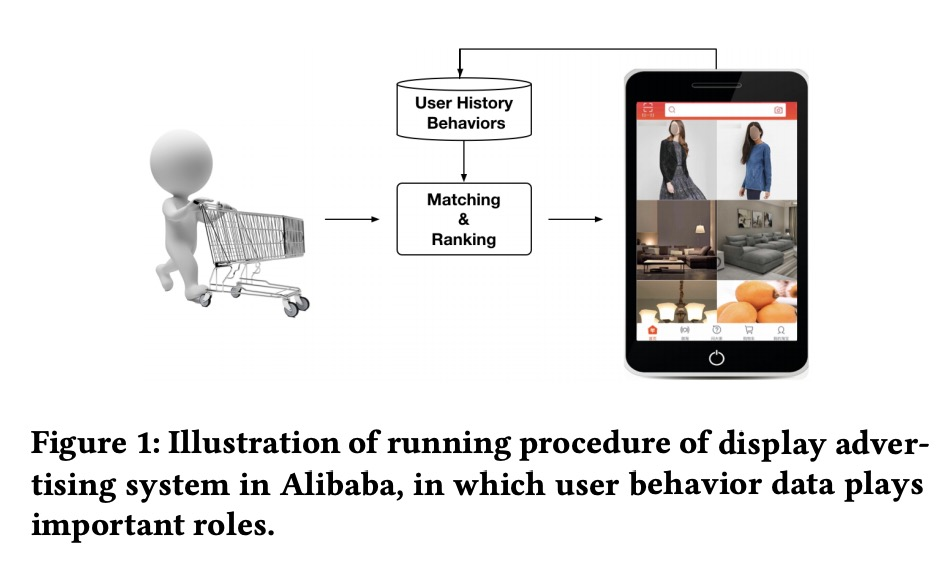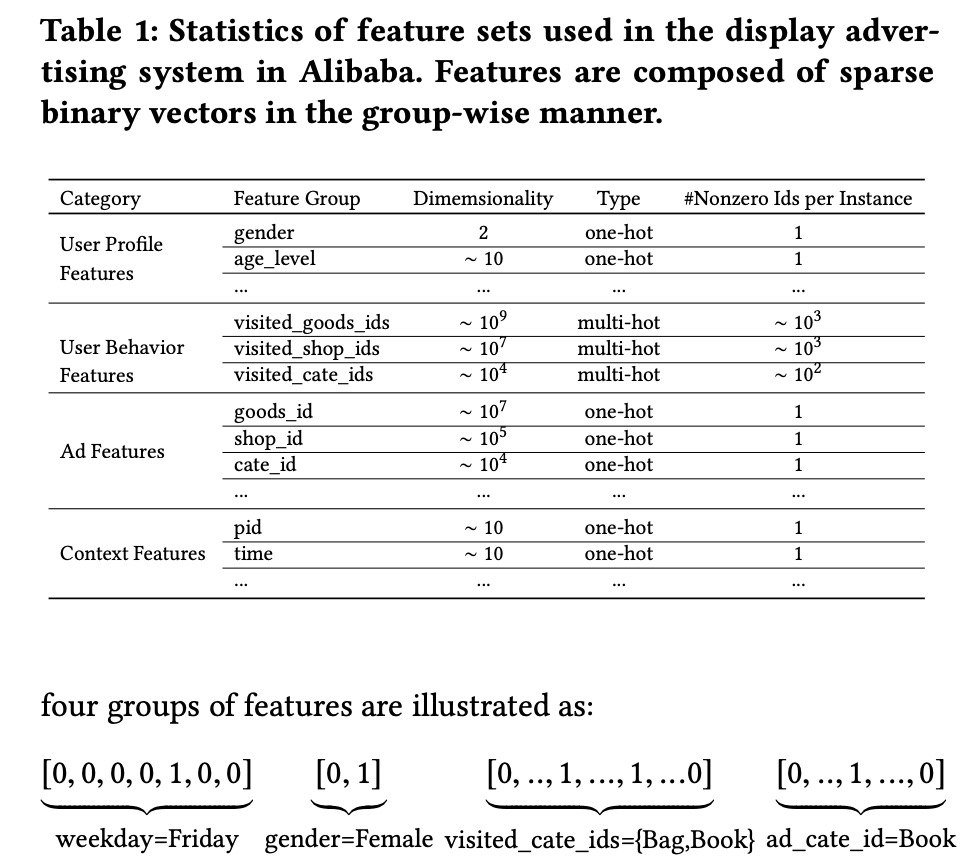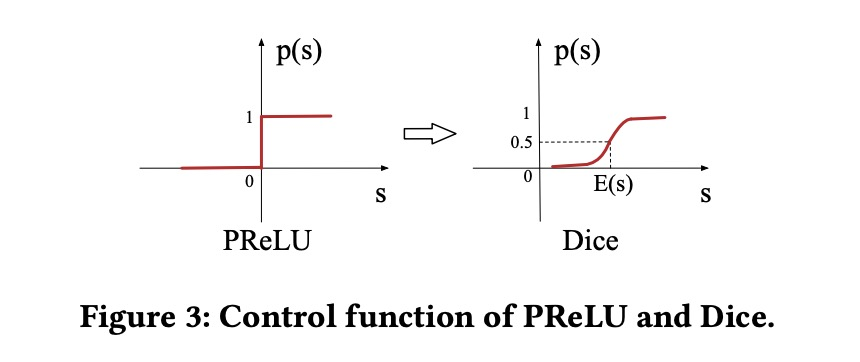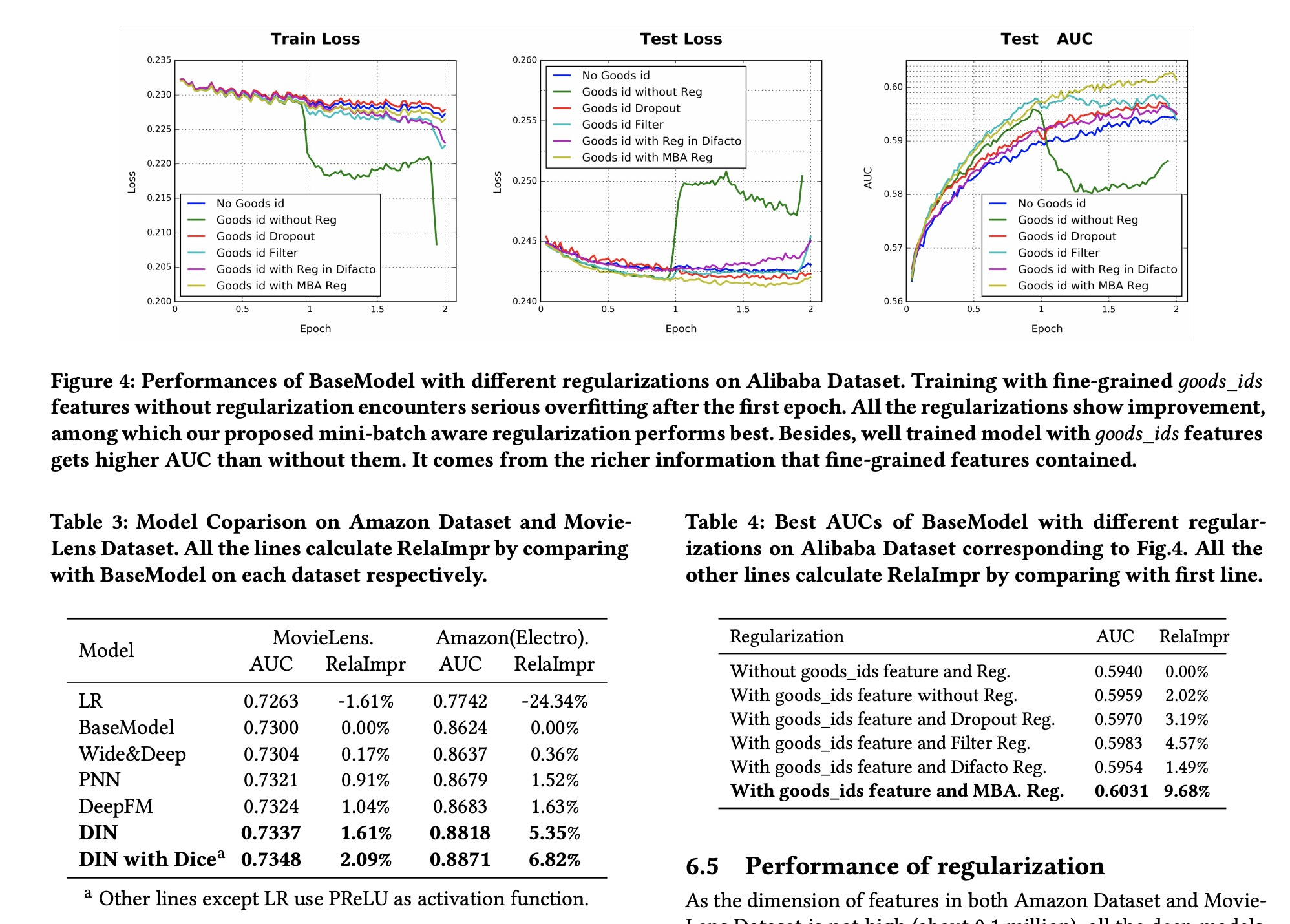# Deep Interest Network for Click-Through Rate Prediction

Posted by maple Blog on April 3, 2019

## Deep Interest Network for Click-Through Rate Prediction

Embedding&MLP

• 学习用户的历史行为喜好，来构建网络，而不是固定大小的vectors来表达
• 为了减少计算量，提出了自适应的正则，只针对非0feature的的部分mini batch做正则计算

• 使用不一样的的激活函数，PReLU和Dicestage 1 ：根据协同过滤产生一些候选集合

stage 2 ：ranking stage，对候选集预估CTR

### Feature Representation### Base Model(Embedding&MLP) 、Deep Interest Network

2者比较，DIN多了个activation Unit 。它的输入为用户历史行为相关的Goods vector和Candidate Ad vectors。用来学习用户历史行为与候选集合的关系。

activation Unit的输出：

$v_U(A)=f(v_U,e_1,e_2,...e_H)=\sum_{j=1}^{H}a(e_j,v_A)e_j=\sum_{j=1}^{H}w_je_j$

### Mini-batch Aware Regularization

$L_2(W)=||W||_2^2=\sum_{j=1}^K||w_j||_2^2=\sum_{(x,y)\in S}\sum_{j=1}^{K}\frac {I(x_j\neq 0)}{n_j}||w_j||_2^2$